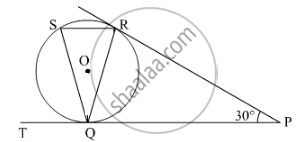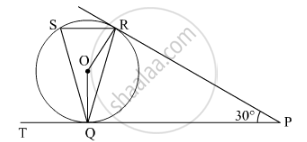# In Fig. 7, tangents PQ and PR are drawn from an external point P to a circle with centre O, such that ∠RPQ = 30°. A chord RS is drawn parallel to the tangent PQ. Find ∠RQS. - Mathematics

In Fig. 7, tangents PQ and PR are drawn from an external point P to a circle with centre O, such that ∠RPQ = 30°. A chord RS is drawn parallel to the tangent PQ. Find ∠RQS.#### SolutionWe know that tangents from an external point are equal in length.

PQ = PR

In PQR,

PQ = PR

PQR=PRQ        (Angles opposite to equal sides are equal.)

Now in ∆PQR,

PQR+PRQ+RPQ=180°

2PQR=180°30°=150°

PQR=75

Also, radius is perpendicular to the tangent at the point of contact.
OQP = ORP = 90°

Now, in PQOR,

RPQ + ORP + OQP + QOR = 360°

⇒ 30° + 90° + 90° + ∠QOR = 360°

⇒ ∠QOR = 360° − 210° = 150°

Since, angle subtended by an arc at any point on the circle is half the angle subtended at the centre by the same arc.

:./_QSR=150^@/2=75^@

Also, QSR = SQT     (Alternate interior angles)

SQT = 75°

Now,

SQT + RQS + PQR = 180°

⇒ 75° + RQS + 75° = 180°

RQS = 180° − 150°

= 30°

Concept: Concept of Circle - Centre, Radius, Diameter, Arc, Sector, Chord, Segment, Semicircle, Circumference, Interior and Exterior, Concentric Circles
Is there an error in this question or solution?
2014-2015 (March) Delhi Set 1
Share